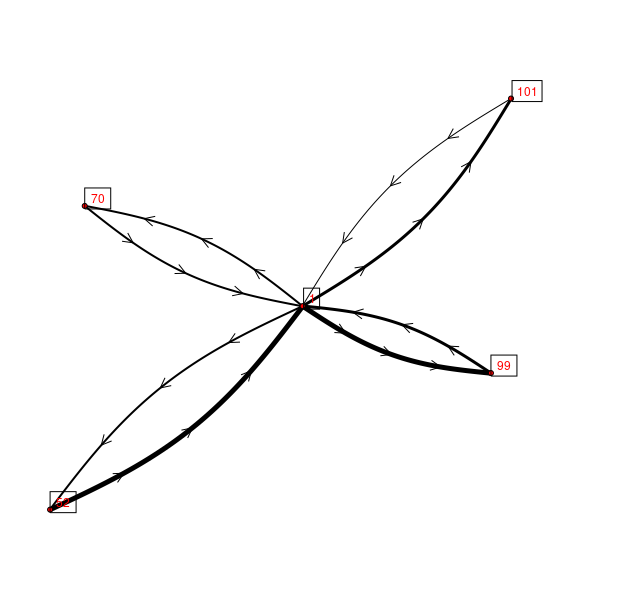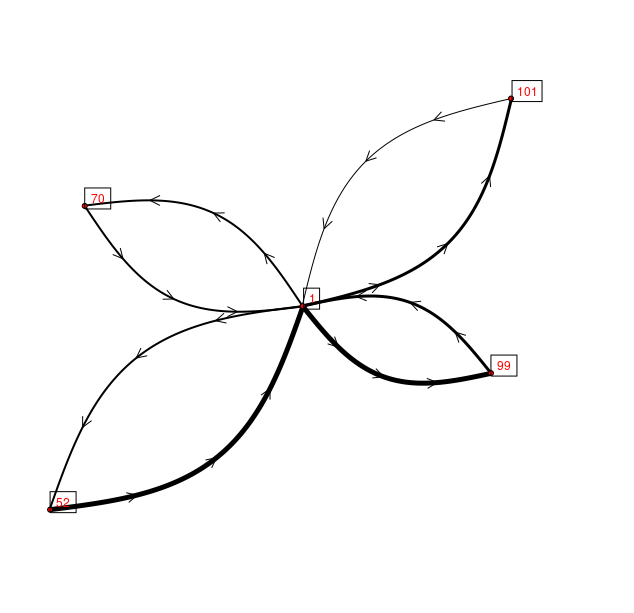## NAME

v.net.curvedarcs - Draws curved arcs between points (e.g. flows)

## KEYWORDS

vector, network, flows

## SYNOPSIS

v.net.curvedarcs
v.net.curvedarcs --help
v.net.curvedarcs [-s] input=name [layer=string] flow_input_file=name [separator=character] output=name minimum_offset=float maximum_offset=float [vertices=integer] [--overwrite] [--help] [--verbose] [--quiet] [--ui]

### Flags:

-s
Draw also short line for flow from and to same node
--overwrite
Allow output files to overwrite existing files
--help
Print usage summary
--verbose
Verbose module output
--quiet
Quiet module output
--ui
Force launching GUI dialog

### Parameters:

input=name [required]
Point map containing origins and destinations
Or data source for direct OGR access
layer=string
Layer number where to find point categories
Vector features can have category values in different layers. This number determines which layer to use. When used with direct OGR access this is the layer name.
Default: 1
flow_input_file=name [required]
File containing origins, destinations and flow volumes
Name of input file
separator=character
Separator used in input text file
Special characters: pipe, comma, space, tab, newline
Default: pipe
output=name [required]
Output map with curved lines
Name for output vector map
minimum_offset=float [required]
minimum offset at furthest point from straight line
maximum_offset=float [required]
maximum offset at furthest point from straight line
vertices=integer
number of vertices used to draw curved lines
Default: 30

## DESCRIPTION

v.net.curvedarcs is a frontend to v.net and v.segment that creates curved arcs between given start and end points. The user provides a point vector map and a text file (see Notes section) with start and end point categories as well as a flow volume for this link which will be transferred to the attribute table of the curved arcs.

The user can determine the nature of the curve with the minimum_offset and maximum_offset parameters. The longest curve's maximum offset from a straight line is equal to the value of maximum_offset in map units. The other curves maximum offset from a straight line is calulated as length(x)/max_length * max_offset. In other words, shorter lines are less curved than longer lines. However, any line's maximum offset is always at least minimum_offset from the straight line.

## EXAMPLE

TODO
```v.extract census_wake2000 cat=1,52,70,99,101 type=centroid out=tmp
v.type tmp from=centroid to=point out=points
echo >> flows.txt << EOF
> from,to,volume
> 52,1,100
> 1,52,50
> 70,1,50
> 1,70,50
> 99,1,66
> 1,99,100
> 101,1,25
> 1,101,75
> EOF
v.net.curvedarcs in=points flow=flows.txt sep=comma out=flows minoff=0 maxoff=2000
d.vect map=flows display=shape,dir width_column=volume width_scale=0.05 size=10
d.vect map=points display=shape,cat fill_color=red icon=basic/circle label_bcolor=black label_size=12 yref=bottom
```The curved arcs with minimum offset = 150 and maximum offset = 2000

Using different amount of curvature:

```v.net.curvedarcs in=points flow=flows.txt sep=comma out=flows minoff=150 maxoff=5000 --o
d.vect map=flows display=shape,dir width_column=volume width_scale=0.05 size=10
d.vect map=points display=shape,cat fill_color=red icon=basic/circle label_bcolor=black label_size=12 yref=bottom
```The curved arcs with minimum offset = 0 and maximum offset = 5000

v.net, v.segment

## AUTHOR

Moritz Lennert, DGES-ULB

## SOURCE CODE

Available at: v.net.curvedarcs source code (history)

Latest change: Thursday Feb 03 09:32:35 2022 in commit: f17c792f5de56c64ecfbe63ec315307872cf9d5c

© 2003-2022 GRASS Development Team, GRASS GIS 8.2.0 Reference Manual